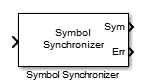# Symbol Synchronizer

Correct symbol timing clock skew

• Library:
• Communications Toolbox / Synchronization

•## Description

The Symbol Synchronizer block corrects symbol timing clock skew for PAM, PSK, QAM, or OQPSK modulation schemes between a single-carrier transmitter and receiver. For more information, see Symbol Synchronization Overview.

Note

The input signal operates on a sample rate basis, while the output signal operates on a symbol rate basis.

## Ports

### Input

expand all

Input samples, specified as a scalar or column vector of a PAM, PSK, QAM, or OQPSK modulated single-carrier signal. This port in unnamed on the block.

Data Types: `double` | `single`
Complex Number Support: Yes

### Output

expand all

Output signal symbols, returned as a variable-size scalar or column vector that has the same data type as the input. For an input with dimensions of Nsamp-by-1, the output at has dimensions of Nsym-by-1. Nsym is approximately equal to Nsamp divided by the Nsps. Nsps is equal to the parameter value. The output length is truncated if it exceeds the maximum output size of $⌈\frac{{N}_{\text{samp}}}{{N}_{\text{sps}}}×1.1⌉$.

This port is unnamed when Normalized timing error output port is not selected.

Estimated timing error for each input sample, returned as a scalar or column vector with values in the range [0, 1]. The estimated timing error is normalized by the input sample time. Err has the same data type and size as the input signal.

#### Dependencies

To enable this port, select Normalized timing error output port.

## Parameters

expand all

Modulation type, specified as `PAM/PSK/QAM`, or `OQPSK`.

Type of timing error detector, specified as ```Zero-Crossing (decision-directed)```, ```Gardner (non-data-aided)```, ```Early-Late (non-data-aided)```, or ```Mueller-Muller (decision-directed)```. This parameter assigns the timing error detection scheme used in the synchronizer.

Samples per symbol, specified as a positive integer greater than 1. For more information, see Nsps in Loop Filter.

Data Types: `double`

Damping factor of the loop filter, specified as a positive scalar. For more information, see ζ in Loop Filter.

Tunable: Yes

Data Types: `double` | `single`

Normalized bandwidth of the loop filter, specified as a positive scalar less than 1. The loop bandwidth is normalized by the sample rate of the input signal. For more information, see BnTs in Loop Filter.

Note

To ensure that the symbol synchronizer locks, set the Normalized loop bandwidth parameter to a value less than `0.1`.

Tunable: Yes

Data Types: `double` | `single`

Phase detector gain, specified as a positive scalar. For more information, see Kp in Loop Filter.

Tunable: Yes

Data Types: `double` | `single`

Select this parameter to output normalized timing error data at the output port Err.

Type of simulation to run, specified as `Code generation` or `Interpreted execution`.

• `Code generation` –– Simulate the model by using generated C code. The first time you run a simulation, Simulink® generates C code for the block. The C code is reused for subsequent simulations unless the model changes. This option requires additional startup time, but the speed of the subsequent simulations is faster than `Interpreted execution`.

• `Interpreted execution` –– Simulate the model by using the MATLAB® interpreter. This option requires less startup time than the `Code generation` method, but the speed of subsequent simulations is slower. In this mode, you can debug the source code of the block.

## Block Characteristics

 Data Types `double` | `single` Multidimensional Signals `no` Variable-Size Signals `yes`